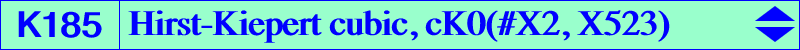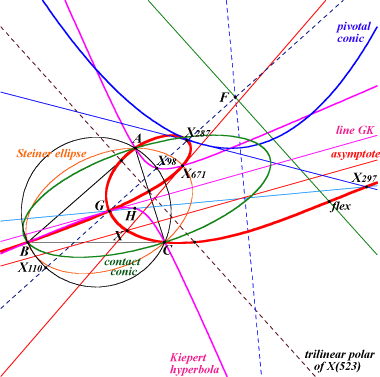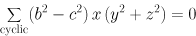X(2), X(287), X(297), X(524), X(671), X(694), X(3978), X(6542), X(6650), X(11078), X(11092), X(17790), X(17946), X(17947), X(17948), X(17949), X(17950), X(17951), X(17952), X(17953) points at infinity of the Steiner ellipse traces of the trilinear polar of X(523) = line X(115)-X(125) through the centers of the Kiepert and Jerabek hyperbolas. cevian O-points, see Table 39.K185 is an isotomic conico-pivotal cubic (see Special Isocubics §8) with root X(523) and node X(2). It is a member of the class CL031 of cubics and probably the most interesting one. It is also a tripolar centroidal cubic of the class CL045. The nodal tangents are parallel to the asymptotes of the Kiepert hyperbola. The pivotal conic is the Kiepert parabola of the antimedial triangle with directrix the Euler line and focus F the anticomplement of X(110). The contact conic is the circum-conic with perspector X(620). The real inflexion point lies on the parallel at X(690) to the trilinear polar of the root X(523) which is the polar line of G in the pivotal conic. K185 is very comparable to the isogonal strophoids K086 (although it is not a strophoid) and specially to the Pelletier strophoid K040, locus of foci of inscribed conics whose center is a point on the line IK = X(1)X(6) or, equivalently, locus of point M such that the midpoint of M and its isogonal conjugate M* lies on the line IK. K185 is indeed the locus of point M such that the midpoint of M and its isotomic conjugate M' lies on the line GK. It is also the transform of the Kiepert hyperbola under the Hirst transformation with pole G with respect to the Steiner circum-ellipse. In barycentric coordinates, this is the mapping (x : y : z) –> (x^2 - y z : y^2 - z x : z^2 - x y). Hence, K185 contains all the images of the points of the Kiepert hyperbola under this transformation, most of them not mentioned in ETC. Furthermore, two antipodes P, Q on the Kiepert hyperbola are transformed into two isotomic conjugates P', Q' on K185, and, obviously, the line P'Q' is tangent to the pivotal conic. For example, X(4) and X(98) give the points X(297) and X(287). See the related K296, K953. K185 belongs to the pencil of cK(#G, P) with P on the trilinear polar of X(598) i.e. the line passing through X(523) and X(351) = center of the Parry circle. These are the only cK(#G, P) with rectangular nodal tangents at G. K185 is the only cK0 of the pencil. The pivotal conics are all inscribed in the antimedial triangle and are centered on the trilinear polar of the isotomic conjugate of X(1992), the reflection of G in K. K185 and K018 generate a pencil of nK0s with same root X(523) and pole on the line X(2)X(6). Each cubic of the pencil is invariant under the involution KW described in Table 62. K185 is the isogonal transform of K222. See the related cubic K381 = cK(#X6, X523) and also K018, locus property 15. See here for a family of related cubics. The barycentric product X(98) × K185 is the Lemoine cubic K1325.